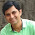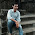## Friday, 25 March 2016

### TILED Matrix Multiplication Using Shared Memory in CUDATiled Matrix Multiplication in CUDA
Today, I am going to discuss Matrix Multiplication in CUDA. In CUDA, number of memories are present. As we have already discussed about the same in previous post "What is CUDA". Matrix Multiplication is very basic but a crucial algorithm in the field of Engineering & Computer Science. I assumed that one who is reading this post knows how to perform Matrix Multiplication in at least one programming language. (C, C++, Python, etc).

Following are the possible number of ways to perform Matrix Multiplication in CUDA.

1. Simple Matrix Multiplication in CUDA.
2. Matrix Multiplication in CUDA by using Shared Memory.
3. Matrix Multiplication in CUDA by using Shared & Constant Memory.
4. Matrix Multiplication in CUDA by using TILES.
5. TILED Matrix Multiplication in CUDA by using Shared & Constant Memory.

The above sequence is arranged in the increasing order of efficiency & performance, 1st being the slowest and 5th is the most efficient & fastest.

One may be confused after reading all these possible number of ways to implement Matrix Multiplication in CUDA and must be wondering that what is the difference between all these ways. Let me tell you that there are Global Memory, Constant Memory, Texture Memory, Shared Memory and Registers present in CUDA. One can use at least one or all of them to implement the algorithm. Just kip in mind that Global Memory is the slowest and Registers are the fastest memory in CUDA. So, it is obvious that if you use faster memory you get output fast. The same statement can be said as if Latency of the memory is less then it gives output quickly.

Go through the following code to understand the algorithm used in the TILED Matrix Multiplication using Shared Memory. You can also get it from my GitHub repository.

```#include <stdio.h>
#include <cuda.h>
#include <stdlib.h>
#define Tile_size 2

//Function To handle any errors occurred in the function calls
#define funcCheck(stmt) do {
cudaError_t err = stmt;
if (err != cudaSuccess) {
printf( "Failed to run stmt %d ", __LINE__);
return -1;
}
} while(0)

int numARows;   // number of rows in the matrix A
int numAColumns;  // number of columns in the matrix A
int numBRows;   // number of rows in the matrix B
int numBColumns;  // number of columns in the matrix B
int numCRows;  // number of rows in the matrix C (you have to set this)
int numCColumns; // number of columns in the matrix C (you have to set this)

// Compute C = A * B
//*************************************************************
//Kernel for shared memory/ Tiled execution
__global__ void matrixMultiplyShared(float * A, float * B, float * C,
int numARows, int numAColumns,
int numBRows, int numBColumns,
int numCRows, int numCColumns)
{
__shared__ float sA[Tile_size][Tile_size];   // Tile size to store elements in shared memory
__shared__ float sB[Tile_size][Tile_size];

int Col = blockDim.x*blockIdx.x + threadIdx.x;
float Cvalue = 0.0;

for (int k = 0; k < (((numAColumns - 1)/ Tile_size) + 1); k++)
{
if ( (Row < numARows) && (threadIdx.x + (k*Tile_size)) < numAColumns)//Copy Data to Tile from Matrix (Global Memory to Shared Memory)
{
}
else
{
}
if ( Col < numBColumns && (threadIdx.y + k*Tile_size) < numBRows)//Copy Data to Tile from Matrix (Global Memory to Shared Memory)
{
}
else
{
}

for (int j = 0; j < Tile_size; ++j)//Multiplying Elements present in tile
{
}
}
if (Row < numCRows && Col < numCColumns)//Saving Final result into Matrix C
{
C[Row*numCColumns + Col] = Cvalue;
}
}
//*************************************************************
void Print_Mat(int Row,int Col,float * Mat)//Function To print the Matrix
{
for(int i=0;i<Row*Col;i++)
{
printf("%f  ",*(Mat+i));

if((i%Col)==0 )
{
printf("\n");
}
}
}//Function close
//*************************************************************
//Normal CPU Matrix Multiplication
void matMultiplyOnHost(float * A, float * B, float * C, int numARows,
int numAColumns, int numBRows, int numBColumns,
int numCRows, int numCColumns)
{
for (int i=0; i < numARows; i ++)
{
for (int j = 0; j < numAColumns; j++)
{
C[i*numCColumns + j ] = 0.0;
for (int k = 0; k < numCColumns; k++)
{
C[i*numCColumns + j ] += A[i*numAColumns + k] * B [k*numBColumns + j];
}
}
}
return;
}
//*************************************************************
int main(int argc, char ** argv) {
float * hostA; // The A matrix
float * hostB; // The B matrix
float * hostC; // The output C matrix
float * hostComputedC;
float * deviceA;
float * deviceB;
float * deviceC;

printf("\nPlease Enter Rows and Columns of A:");
scanf("%d %d",&numARows,&numAColumns);

printf("\nPlease Enter Rows and Columns of B:");
scanf("%d %d",&numBRows,&numBColumns);

hostA = (float *) malloc(sizeof(float)*numARows*numAColumns);
hostB = (float *) malloc(sizeof(float)*numBRows*numBColumns);

for (int i = 0; i < numARows*numAColumns; i++)//Matrix Initialization
{
hostA[i]=1.0;
}
for (int i = 0; i < numBRows*numBColumns; i++)
{
hostB[i]=1.0;
}

printf("\nMatrix A Values:\n");
Print_Mat(numARows,numAColumns,hostA);//Function Call

printf("\n\nMatrix B Values:\n");
Print_Mat(numBRows,numBColumns,hostB);//Function Call

// Setting numCRows and numCColumns
numCRows = numARows;
numCColumns = numBColumns;

hostC = (float *) malloc(sizeof(float)*numCRows*numCColumns);
hostComputedC = (float *) malloc(sizeof(float)*numCRows*numCColumns);

// Allocating GPU memory
funcCheck(cudaMalloc((void **)&deviceA, sizeof(float)*numARows*numAColumns));
funcCheck(cudaMalloc((void **)&deviceB, sizeof(float)*numBRows*numBColumns));
funcCheck(cudaMalloc((void **)&deviceC, sizeof(float)*numCRows*numCColumns));

// Copy memory to the GPU
funcCheck(cudaMemcpy(deviceA, hostA, sizeof(float)*numARows*numAColumns, cudaMemcpyHostToDevice));
funcCheck(cudaMemcpy(deviceB, hostB, sizeof(float)*numBRows*numBColumns, cudaMemcpyHostToDevice));

// Initialize the grid and block dimensions

dim3 dimGrid((numCColumns/Tile_size) + 1, (numCRows/Tile_size) + 1, 1);//Number of Blocks required
dim3 dimBlock(Tile_size, Tile_size, 1);//Number of threads in each block

//@@ Launch the GPU Kernel here
matrixMultiplyShared<<<dimGrid, dimBlock>>>(deviceA, deviceB, deviceC, numARows, numAColumns, numBRows, numBColumns, numCRows, numCColumns);

cudaError_t err1 = cudaPeekAtLastError();//To capture last error in function call

// Copy the results in GPU memory back to the CPU
funcCheck(cudaMemcpy(hostC, deviceC, sizeof(float)*numCRows*numCColumns, cudaMemcpyDeviceToHost));

printf("\nMatrix C From Device\n");
Print_Mat(numCRows,numCColumns,hostC);//Function Call

matMultiplyOnHost(hostA, hostB, hostComputedC, numARows, numAColumns, numBRows, numBColumns, numCRows, numCColumns);

printf("\nMatrix C From Host\n");
Print_Mat(numCRows,numCColumns,hostComputedC);//Function Call

for (int i=0; i < numCColumns*numCRows; i++)//Compare both the result matrices 1. MatrixMultiplyonHost 2. MatrixMultiplyonDevice
{
if (hostComputedC[i]  != hostC[i] )
{
printf("Mismatch at Row = %d Col = %d hostComputed[] = %f --device[] %f\n", i / numCColumns, i % numCColumns, hostComputedC[i], hostC[i]);
break;
}
}

printf("\n Number of Blocks Created:%d \n",((numCColumns/Tile_size) + 1)*((numCColumns/Tile_size) + 1));
printf("\n Number of Threads Per Block: %d \n",(Tile_size*Tile_size));

// Free the GPU memory
funcCheck(cudaFree(deviceA));
funcCheck(cudaFree(deviceB));
funcCheck(cudaFree(deviceC));
//Free the Pointer Memory
free(hostA);
free(hostB);
free(hostC);
free(hostComputedC);

return 0;
}```

==>Posted By Yogesh B. Desai

Next Post: Parallel Programming Section

Previous Post: Vector Arithmetic Operations in CUDA

1.1.